# Analysis: Checking Raval and Ramanathan

A seminal paper from Raval and Ramanathan (1989)1The original paper was published in Nature in 1989, but is behind a paywall. Fortunately, it was reprinted in a conference proceeding soon after; that’s the link I’ve offered. defines the normalized greenhouse effect,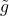, and indicates that, in the absence of lapse rate or water vapor feedbacks,wouldn’t be expected to change as the surface temperature increases. In this analysis, I investigate their mathematical analysis to try to understand what if any assumptions are involved.

## Their key equation

R&R present the following radiative transfer equation (TRE):

(1)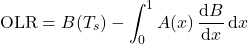where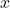is the pressure divided by surface pressure; the blackbody emission is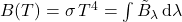; and the effective absorptivity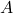is the “integral of the monochromatic absorptivityweighted with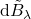, and normalized by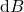.”2For this, R&R cite Manabe & Wetherald (1967). However, I don’t see the equation in M&W (1967). “Thus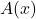is the absorptivity between the TOA (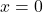) and the pressure level.”

Let’s try transforming the prior integral using integration by parts:

(2)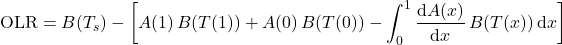(3)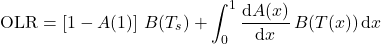## Schwarzchild’s equation of radiative transfer

Let’s try to see how the above relates to Scharzchild’s equation of radiative transfer (which governs propagation of thermal radiation in the absence of scattering):

(4)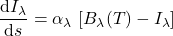where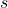is distance in a particular direction and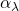is the wavelength-dependent absorption coefficient. This equation has the solution

(5)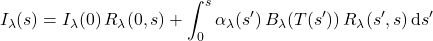where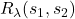is the transmittance between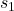and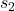,

(6)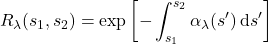If we define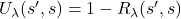, then

(7)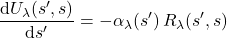Thus integration by parts leads to:

(8)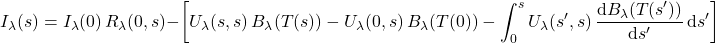Applying the definition of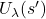leads to:

(9)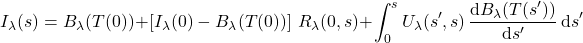If we assume the surface air temperature matches the surface temperature and the surface is a blackbody, this becomes:

(10)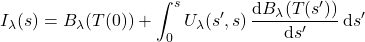Now let’s change this to a more useful coordinate, by setting. This leads to:

(11)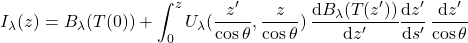(12)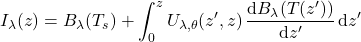where

(13)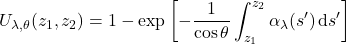Now let’s change variables again, to use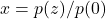where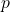is pressure. This leads to:

(14)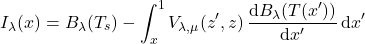where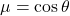and

(15)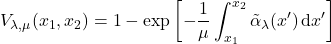(16)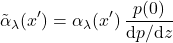To get from intensity to flux, we need to integrate over all directions (within the upward hemisphere of solid angles). This involves an integration of the form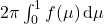where. This leads to:

(17)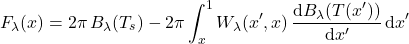where

(18)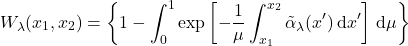If we define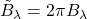,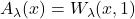, and compute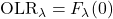, and this becomes

(19)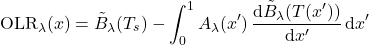We can integrate this over wavelength,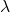, and obtain R&R’s version of the RTE, but only if we assume that the absorption coefficient,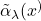is independent of wavelength, i.e., we have a “gray” atmosphere.

## Conclusions

Thus, the result thatwill not change as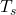varies depends on these assumptions:

• Negligible scattering (as is implicit in Schwarzchild equation)
• Fixed lapse rate (as R&R name)
• Fixed atmospheric composition (including absolute humidity)
• Temperature varies linearly with altitude and pressure varies exponentially with altitude
• Surface is a blackbody with a temperature that matches the surface atmospheric temperature
• The atmospheric absorption/emission is “gray”, i.e., independent of wavelength